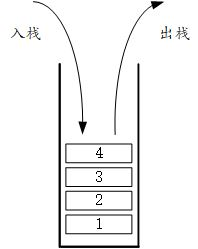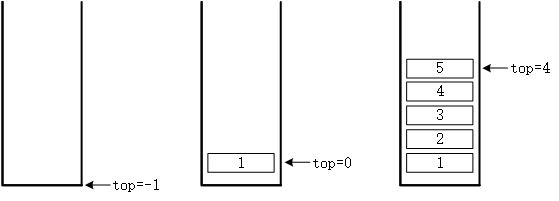## 栈的概念## 实现

int maxSize = 100;
int top = -1;
int* stack = new int[maxSize];


top是一个int类型的值，称之为栈中的头指针或者栈顶。top永远指向当前栈中存储的数据的顶部，在初始化时，栈中是空的，所以我们将top值设为1 。可以发现，当top = -1时表示空栈，也就是栈里没有数据，当top >= 0时，即表示当前栈顶的元素为stack[top]。top++;
stack[top] = value;


int value = stack[top];


top指向的也就是栈顶的值

int value = stack[top];
top--;


value即是出栈前，栈顶的元素。出栈时我们并不需要手动的再将原先的元素在数组中的数据清空，只需要简单的修改top指针指向的位置，在下一次入栈时原先的数据将会被直接覆盖。同样的道理，清空整个栈也只需要将top设为-1就可以了。

top = -1; // 清空栈


## 完整代码

//顺序栈
template <class Type>
class ArrayStack {
private:
// 栈顶指针
int top;
// 栈的最大容量
int maxSize;
// 数据指针
Type* date;

public:
// 构造函数，默认创建大小为100的顺序栈
ArrayStack();
// 构造函数，创建大小为max的顺序栈
ArrayStack(int max);
// 析构函数，释放空间
~ArrayStack();
// 入栈，成功返回true，失败返回false
bool push(Type d);
// 出栈，返回出栈前栈顶的元素
Type pop();
// 获取栈顶元素
Type getTop();
// 获取当前栈的空间
int getSize();
// 判断空栈
bool isEmpty();
// 清空栈中的元素
void setEmpty();
};
template <class Type>
ArrayStack<Type>::ArrayStack() {
top = -1;
maxSize = 100;
date = new Type[maxSize];
}
template <class Type>
ArrayStack<Type>::ArrayStack(int max) {
top = -1;
maxSize = max;
date = new Type[maxSize];
}
template <class Type>
ArrayStack<Type>::~ArrayStack() {
top = -1;
delete date;
date = nullptr;
}
template <class Type>
bool ArrayStack<Type>::push(Type d) {
if (top + 1 == maxSize) {
return false;
}

top++;
date[top] = d;
return true;
}
template <class Type>
Type ArrayStack<Type>::pop() {
if (top == -1) {
return false;
}

Type temp = date[top];
top--;
return temp;
}
template <class Type>
Type ArrayStack<Type>::getTop() {
if (top == -1) {
return false;
}

Type temp = date[top];
return temp;
}
//返回当前长度 top+1
template <class Type>
int ArrayStack<Type>::getSize() {